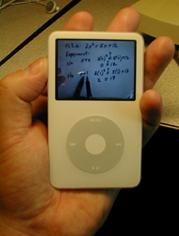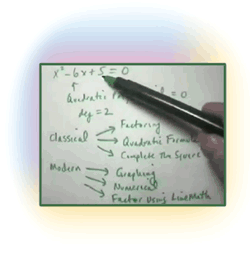# Distance Precalculus with Trigonometry

Precalculus is the course before the main Calculus sequence, and is known by many different names:

• in most U.S. high schools: Trigonometry + Math Analysis
• in many U.S. colleges: College Algebra + Trigonometry

Simply put, Precalculus studies all of the topics of high school algebra: solving equations, graphing, quadratic formula, rational polynomials, factoring, logarithms, exponentials, trigonometry, and additional topics like systems of equations, inequalities, conic sections, et al.

# Course Catalog Listing

MAT 1140: Precalculus with Trigonometry [4 credit hours]

Course Description: Properties of real numbers, order and absolute value, complex numbers, scientific notation, factoring polynomials, linear and quadratic equations, systems of equations, linear inequalities, and graphing, exponential, logarithmic, and trigonometric functions, including identities, inverse trigonometric functions, and right triangle trigonometry.

Prerequisite: Algebra I, II; Geometry

## Fresh, Innovative Approach

There are many Precalculus courses taught on-line throughout the world, which mainly consist of using Blackboard to host the course, problems are assigned from a paper textbook, quizzes are multiple-choice, and you can email your instructor if you have questions.

Our Distance Precalculus is a completely different course.

We do not believe paper textbooks and multiple choice answers are educationally beneficial. Such courses may be easy to "pass", but we do not feel such courses prepare you for future Calculus study, which is the core reason why you take a precalculus course.We are taking Distance math education to the next level: Video study and student video creation, coupled with high-powered computer algebra software, following a dynamic and exciting curriculum.

## Methodologies

Distance Precalculus is taught using the following methodologies:

• Asynchronous Studying
This means that the course happens on your schedule, not at fixed times. You may work as intensely as you wish, take a break for a week or two, and then resume, all on your needs.

• Mastery Learning
The student will learn the current module of material thoroughly before moving on to the next topic. This eliminates the "slippery slope" problem of not mastering a previous topic when faced with a new topic.

• Synchronous Communications
The majority of communcation between the student and the instructors will occur via Chat, so that problems, questions, issues, that come up are solved in real time.

• ChalkTalk Video
Video presentations of problems solved manually, "by hand", providing the basis of the classical study of precalculus topics.

Example Videos

• Computer Algebra Video
Video presentations of problems solved using the computer algebra system LiveMath, providing the basis of the modern study of precalculus topics.• Written Solution Sets
Students will complete written solution sets, scan them to PDF, and turn them in on-line for grading. These problem sets will be from the classical side of the precalculus curriculum - the "math you need to be able to do by hand."

• Video Solution Sets
Students will complete Video Solutions of various problems, and turn these videos in on-line for grading, thus reinforcing the "math you need to be able to do by hand" goal.

• Computer Algebra Notebook Solution Sets
Students will complete notebook questions in using LiveMath software dealing with more modern types of questions in Precalculus requiring technological approaches.

Similar to our Distance Calculus course structure, this course is based upon heavy amounts of communication between the student and the instructional team. All assignments are based upon the mastery learning model, and turned in recursively back-and-forth between student and instructor until 100% mastery is achieved.

This is an excellent course for the computer novice. Even if checking your email is a not a "natural" activity for you, the Distance Precalculus course is an excellent way to simultaneously prepare for Calculus, and conquer whatever computer "phobia" fears you may have. By the end of this course, you won't be nearly as scared of your computer as you had been at the start - you might even like your computer.